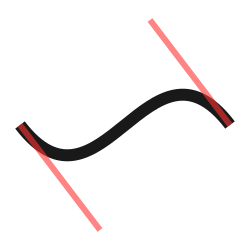# Example scripts¶

This is a set of example scripts from the `examples` directory.

## How curves work¶```"""
Simple path curve example
Adapted from the Cairo samples page <https://www.cairographics.org/samples/>
and annotated based on the Nodebox "How curves work" example
"""
size(250, 250)

# Set up the canvas
# Don't close paths when we call endpath()
autoclosepath(False)
# Don't fill any path
nofill()

# First, let's set the point coordinates.

# Start curve point
x, y = 20, 125
# Left control point
x1, y1 = 100, 230
# Right control point
x2, y2 = 150, 20
# End curve point
x3, y3 = 230, 125

# Note that
#     x, y = 25, 125
# is equivalent to
#     x = 25
#     y = 125
# but this way it's somewhat more elegant.

# Draw the curve
strokewidth(12)
stroke(0.1)
# Start drawing the bezier path
beginpath()
# Move to the starting point
moveto(x, y)
# For curveto(), the first 4 parameters are the coordinates of the two control
# points; the last two parameters are the coordinates of the destination point.
curveto(x1, y1, x2, y2, x3, y3)
# We're done, stop path drawing and render it
endpath()

# To show where the control points are,
# we draw helper lines
strokewidth(6)
stroke(1, 0.2, 0.2, 0.6)
# The first control point starts at the x, y position
line(x, y, x1, y1)
# And the second control point is the end curve point
line(x2, y2, x3, y3)
```